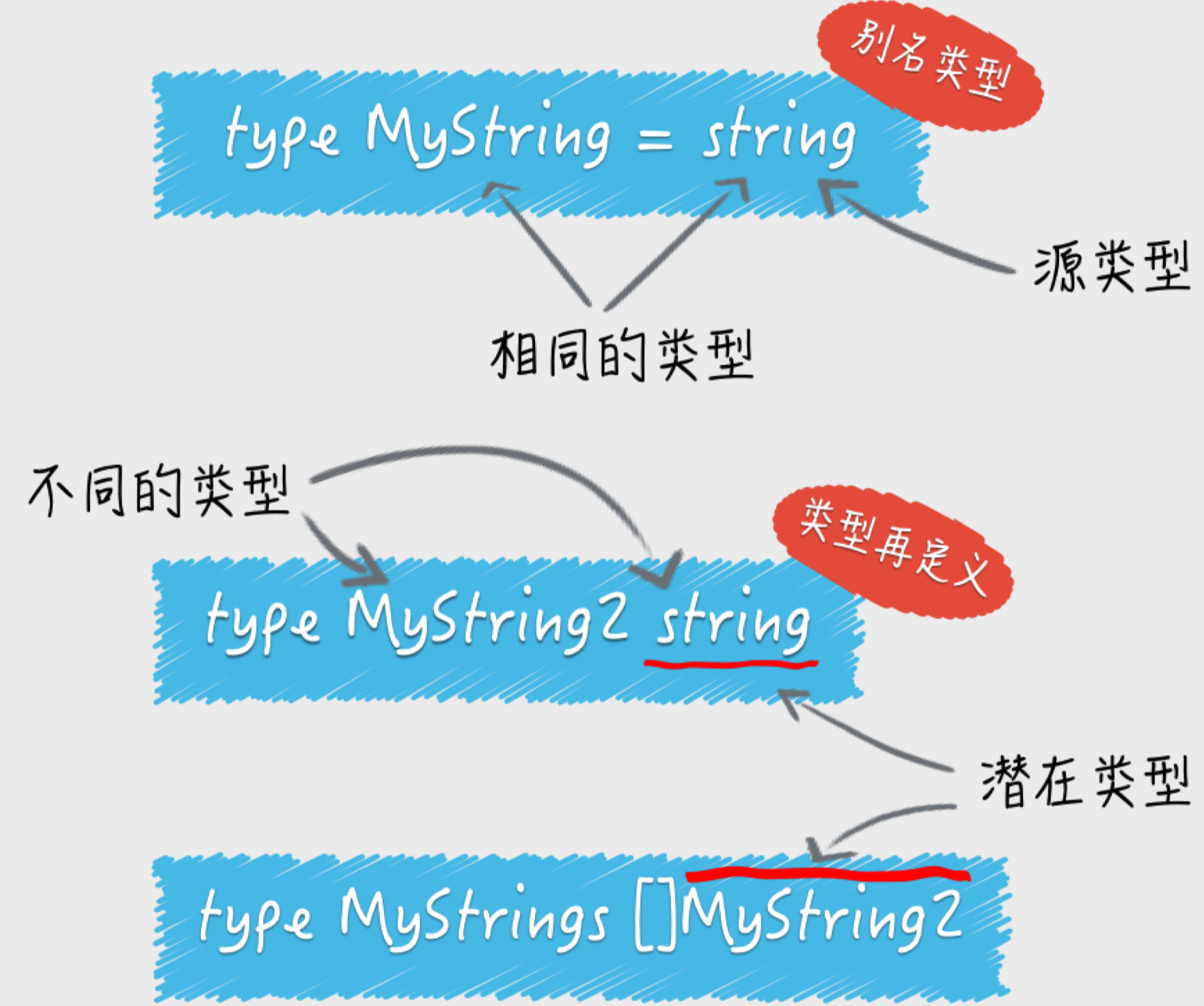# Java程序员学习Go指南（一）

2019/04/10 10:10

## GOPATH 工作空间

GOPATH简单理解成Go语言的工作目录，它的值是一个目录的路径，也可以是多个目录路径，每个目录都代表Go语言的一个工作区（workspace）。

### 源码安装

``````github.com/labstack/echo，
``````

``````go install github.com/labstack/echo
``````

## 代码块中的重名变量

``````var block = "package"

func main() {
block := "function"
{
block := "inner"
fmt.Printf("The block is %s.\n", block)
}
fmt.Printf("The block is %s.\n", block)
blockFun()
}
``````

``````The block is inner.
The block is function.
``````

⼀般情况下，程序会⼀直查到当前代码包代表的代码块。如果仍然找不到，那么Go语⾔的编译器就会报错了。

## 变量的类型

### 判断变量类型

``````func main() {
container := map[int]string{0: "zero", 1: "one", 2: "two"}
fmt.Printf("The element is %q.\n", container)

value2, ok2 := interface{}(container).(map[int]string)
value1,   ok1 := interface{}(container).([]string)
fmt.Println(value1)
fmt.Println(value2)
if !(ok1 || ok2) {
fmt.Printf("Error: unsupported container type: %T\n", container)
return
}
}
``````

### 强制类型转换

``````	var srcInt = int16(-255)
dstInt := int8(srcInt)
fmt.Println(dstInt)
``````

### 类型别名和潜在类型

``````type MyString = string
``````

``````type MyString2 string // 注意，这⾥没有等号。
````````````type MyString string
str := "BCD"
myStr1 := MyString(str)
myStr2 := MyString("A" + str)
``````

``````type MyString2 string
str := "BCD"
myStr2 := MyString2(str)

//myStr2 = str // 这里的赋值不合法，会引发编译错误。

//fmt.Printf("%T(%q) == %T(%q): %v\n",
//	str, str, myStr2, myStr2, str == myStr2)  // 这里的判等不合法，会引发编译错误。
``````

``````type MyString string
strs := []string{"E", "F", "G"}
var myStrs []MyString
//myStrs := []MyString(strs) // 这里的类型转换不合法，会引发编译错误。
``````

## 管道channel

⼀个通道相当于⼀个先进先出（FIFO）的队列。也就是说，通道中的各个元素值都是严格地按照发送的顺序排列的，先被发 送通道的元素值⼀定会先被接收。元素值的发送和接收都需要⽤到操作符<-。我们也可以叫它接送操作符。⼀个左尖括号紧 接着⼀个减号形象地代表了元素值的传输⽅向。

``````func main() {
ch1 := make(chan int, 3)
//往channel中放入元素
ch1 <- 2
ch1 <- 1
ch1 <- 3
//往channel中获取元素
elem1 := <-ch1
fmt.Printf("The first element received from channel ch1: %v\n",
elem1)
}
``````

### 基本特性

1. 对于同⼀个通道，发送操作之间是互斥的，接收操作之间也是互斥的。

1. 发送操作和接收操作中对元素值的处理都是不可分割的。 如发送操作要么还没复制元素值，要么已经复制完毕，绝不会出现只复制了⼀部分的情况。

2. 发送操作在完全完成之前会被阻塞。接收操作也是如此。 发送操作包括了“复制元素值”和“放置副本到通道内部”这两个步骤。

### ⻓时间的阻塞

1. 缓冲通道 如果通道已满，那么对它的所有发送操作都会被阻塞，直到通道中有元素值被接收⾛。

``````	// 示例1。
ch1 := make(chan int, 1)
ch1 <- 1
//ch1 <- 2 // 通道已满，因此这里会造成阻塞。

// 示例2。
ch2 := make(chan int, 1)
//elem, ok := <-ch2 // 通道已空，因此这里会造成阻塞。
//_, _ = elem, ok
ch2 <- 1
``````
1. ⾮缓冲通道

⽆论是发送操作还是接收操作，⼀开始执⾏就会被阻塞，直到配对的操作也开始执⾏，才 会继续传递。由此可⻅，⾮缓冲通道是在⽤同步的⽅式传递数据。也就是说，只有收发双⽅对接上了，数据才会被传递。

``````	ch1 := make(chan int )
ch1 <- 10
fmt.Println("End." )//这里会造成阻塞。
``````

### 关闭通道

``````func main() {
ch1 := make(chan int, 2)
// 发送方。
go func() {
for i := 0; i < 10; i++ {
fmt.Printf("Sender: sending element %v...\n", i)
ch1 <- i
}
fmt.Println("Sender: close the channel...")
close(ch1)
}()

// 接收方。
for {
elem, ok := <-ch1
if !ok {
break
}
}

fmt.Println("End.")
}
``````

### 单向通道

``````var uselessChan = make(chan<- int, 1)
``````

``````func main() {
// 初始化一个容量为3的通道
intChan1 := make(chan int, 3)
//将通道传入到函数中
SendInt(intChan1)
}
//使用单向通道限制这个函数只能放入元素到通道中
func SendInt(ch chan<- int) {
ch <- rand.Intn(1000)
}
``````

``````func main() {
intChan2 := getIntChan()
for elem := range intChan2 {
fmt.Printf("The element in intChan2: %v\n", elem)
}
}

func getIntChan() <-chan int {
num := 5
ch := make(chan int, num)
for i := 0; i < num; i++ {
ch <- i
}
close(ch)
return ch
}
``````

### select多路选择

#### select语句与通道联⽤

select语句只能与通道联⽤，它⼀般由若⼲个分⽀组成。每次执⾏这种语句的时候，⼀般只有⼀个分⽀中的代码会被运⾏。

``````func example1() {
// 准备好几个通道。
intChannels := chan int{
make(chan int, 1),
make(chan int, 1),
make(chan int, 1),
}
// 随机选择一个通道，并向它发送元素值。
index := rand.Intn(3)
fmt.Printf("The index: %d\n", index)
intChannels[index] <- index
// 哪一个通道中有可取的元素值，哪个对应的分支就会被执行。
select {
case <-intChannels:
fmt.Println("The first candidate case is selected.")
case <-intChannels:
fmt.Println("The second candidate case is selected.")
case elem := <-intChannels:
fmt.Printf("The third candidate case is selected, the element is %d.\n", elem)
default:
fmt.Println("No candidate case is selected!")
}
}
``````

1. 如果像上述示例那样加⼊了默认分⽀，那么⽆论涉及通道操作的表达式是否有阻塞，select语句都不会被阻塞。如果那 ⼏个表达式都阻塞了，或者说都没有满⾜求值的条件，那么默认分⽀就会被选中并执⾏。
2. 如果没有加⼊默认分⽀，那么⼀旦所有的case表达式都没有满⾜求值条件，那么select语句就会被阻塞。直到⾄少有⼀ 个case表达式满⾜条件为⽌。
3. select语句只能对其中的每⼀个case表达式各求值⼀次。
4. select语句包含的候选分⽀中的case表达式都会在该语句执⾏开始时先被求值，并且求值的顺序是依从代码编写的顺序 从上到下的。
5. 对于每⼀个case表达式，如果其中的发送表达式或者接收表达式在被求值时，相应的操作正处于阻塞状态，那么对 该case表达式的求值就是不成功的。
6. 如果select语句发现同时有多个候选分⽀满⾜选择条件，那么它就会⽤⼀种伪随机的算法在这些分⽀中选择⼀个并执⾏。

#### 超时控制

select 里面会根据两个case的返回时间来选择运行，哪个先返回哪个就先执行，所以利用这个功能，可以实现超时返回。

``````func TestSelect(t *testing.T) {
//select 里面会根据两个case的返回时间来选择运行
//哪个先返回哪个就先执行
//所以利用这个功能，可以实现超时返回
select {
case ret:=<-AsyncService():
t.Log(ret)
case <-time.After(time.Microsecond*100):
t.Error("time out")
}
}

func AsyncService() chan string {
retCh := make(chan string,1)
go func() {
ret := service()
fmt.Println("return result.")
retCh <- ret
fmt.Println("service exited.")
}()
return retCh
}
``````

## 函数

### 接受其他的函数作为参数传⼊

``````type operate func(x, y int) int
``````

``````func calculate(x int, y int, op operate) (int, error) {
if op == nil {
return 0, errors.New("invalid operation")
}
return op(x, y), nil
}
``````

### 闭包

``````type calculateFunc func(x int, y int) (int, error)

func genCalculator(op operate) calculateFunc {
return func(x int, y int) (int, error) {
if op == nil {
return 0, errors.New("invalid operation")
}
return op(x, y), nil
}
}

func main() {
x, y = 56, 78
fmt.Printf("The result: %d (error: %v)\n",
result, err)
}
``````

### 参数值在函数中传递

1. 值类型 所有传给函数的参数值都会被复制，函数在其内部使⽤的并不是参数值的原 值，⽽是它的副本。

``````func main() {
// 示例1。
array1 := string{"a", "b", "c"}
fmt.Printf("The array: %v\n", array1)
array2 := modifyArray(array1)
fmt.Printf("The modified array: %v\n", array2)
fmt.Printf("The original array: %v\n", array1)
fmt.Println()

}

// 示例1。
func modifyArray(a string) string {
a = "x"
return a
}
``````

``````The array: [a b c]
The modified array: [a x c]
The original array: [a b c]
``````

1. 引用类型 对于引⽤类型，⽐如：切⽚、字典、通道，像上⾯那样复制它们的值，只会拷⻉它们本身⽽已，并不会拷⻉它们引⽤的 底层数据。也就是说，这时只是浅表复制，⽽不是深层复制。

``````func main() {
slice1 := []string{"x", "y", "z"}
fmt.Printf("The slice: %v\n", slice1)
slice2 := modifySlice(slice1)
fmt.Printf("The modified slice: %v\n", slice2)
fmt.Printf("The original slice: %v\n", slice1)
fmt.Println()
}
func modifySlice(a []string) []string {
a = "i"
return a
}
``````

``````The slice: [x y z]
The modified slice: [x i z]
The original slice: [x i z]
``````

1. 引用类型和值类型结合的类型

``````func main() {
complexArray1 := []string{
[]string{"d", "e", "f"},
[]string{"g", "h", "i"},
[]string{"j", "k", "l"},
}
fmt.Printf("The complex array: %v\n", complexArray1)
complexArray2 := modifyComplexArray(complexArray1)
fmt.Printf("The modified complex array: %v\n", complexArray2)
fmt.Printf("The original complex array: %v\n", complexArray1)
}

func modifyComplexArray(a []string) []string {
a = "s"
a = []string{"o", "p", "q"}
return a
}
``````

``````The complex array: [[d e f] [g h i] [j k l]]
The modified complex array: [[d e f] [g s i] [o p q]]
The original complex array: [[d e f] [g s i] [j k l]]
``````

0
0 收藏

### 作者的其它热门文章0 评论
0 收藏
0